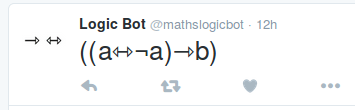# @mathslogicbotNearly a year ago, I made @mathslogicbot, a Twitter bot which is tweeting all logical tautologies in propositional calculus that are less than 140 characters long. This blog post explains what this means.

### What is propositional calculus?

Propositional calculus is a formal system of logic that allows mathematicians to study the properties of the logical systems underlying everything else.In propositional calculus the following symbols are allowed: variables ($a$ to $z$ and $\alpha$ to $\lambda$), not ($\neg$), implies ($\rightarrow$), if and only if ($\leftrightarrow$), and ($\wedge$), or ($\vee$) and brackets ($()$).

Formulae, or logical ‘sentences’, can be build from the symbols. Formulae can be built recursively using the following rules:

• Every variable is a formula.
• If $A$ is a formula, then $\neg A$ is a formula.
• If $A$ and $B$ are formulae then $(A\rightarrow B)$, $(A\leftrightarrow B)$, $(A\wedge B)$, and $(A\vee B)$ are all formulae.

For example, $(a\vee b)$ is a formula, meaning “$a$ or $b$” and $\neg f$ is a formula meaning “not $f$”.

If each of the variables is assigned a value of either “true” or “false”, the truth of each formula can be determined by using the following definitions of the symbols:

• $\neg a$ is true if $a$ is false (and false otherwise).
• $(a\wedge b)$ is true if $a$ and $b$ are both true (and false otherwise).
• $(a\vee b)$ is true if $a$ or $b$ is true (or both are true) (and false otherwise).
• $(a\leftrightarrow b)$ is true if $a$ and $b$ are either both true or both false (and false otherwise).
• ($a \rightarrow\ b)$ is true if $a$ and $b$ are both true or $a$ is false (and false otherwise).

These can be summarised using truth tables:

#### Tautologies

Which of these is the best tautology?

View ResultsLoading ...

A tautology is a formula that is true for any assingment of truth values to the variables. For example, $(a\vee \neg a)$ is a tautology because: if $a$ is true then $\neg a$ is false; if $a$ is false, $\neg a$ is true. In both cases $a$ or $\neg a$, i.e. $(a\vee\neg a)$, is true.

@mathslogicbot works by generating formulae and trying all possible assignments of truth values. If it finds a formula that is true for every assignment, it has found a tautology to tweet. It tweets one of these every three hours.

### A sequence

After setting @mathslogicbot off, I began to wonder how long it would take to finish all the tautologies. To get an idea of this, we can look at the sequence (A256120 on OEIS):
$$a_n = \text{number of tautologies with }n\text{ characters}$$
The first thirteen terms of this sequence are
$$0, 0, 0, 0, 2, 2, 12, 6, 57, 88, 373, 554, 2086$$
In total, there are 3180 tautologies with 13 characters or less. These alone will take 397 days to tweet and the numbers seem to be getting larger as the sequence continues. It looks like we could be waiting for a long time for @mathslogicbot to finish…

If you want to play with tautologies, then you can download the Python source code @mathslogicbot uses to generate them on GitHub.Matthew is a postdoctoral researcher at University College London. He hasn’t had time to play Klax since the noughties, but he’s pretty sure that Coke is it!